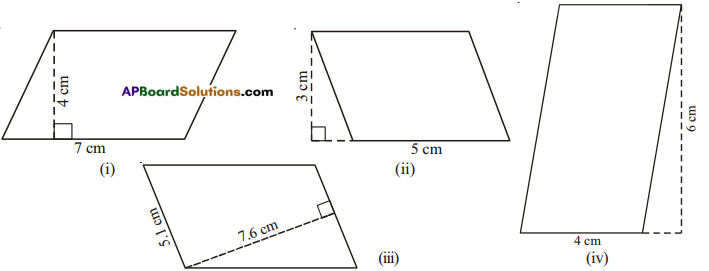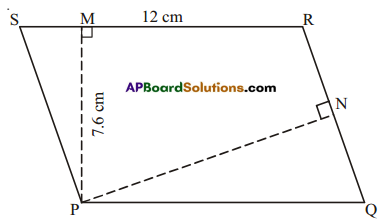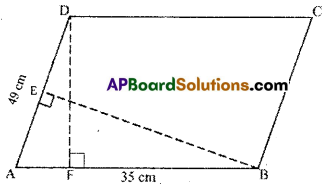# AP Board 7th Class Maths Solutions Chapter 13 Area and Perimeter Ex 2

AP State Syllabus AP Board 7th Class Maths Solutions Chapter 13 Area and Perimeter Ex 2 Textbook Questions and Answers.

## AP State Syllabus 7th Class Maths Solutions 13th Lesson Area and Perimeter Exercise 2Question 1.
Find the area of the each of the following parallelograms.Solution:

 Fig Base Height A = Base x Height (i) 7 4 7 x 4 = 28cm2 (ii) 5 3 5 x 3  = 15 cm2 (iii) 5.1 7.6 5.1 x 7.6 = 38.76 cm2 (iv) 4 6 4 x 6 = 24cm2Question 2.
PQRS is a parallelogram. PM is the height from P to $$\overline{\mathrm{SR}}$$ and PN is the height from P to $$\overline{\mathrm{QR}}$$. If SR = 12cm and PM = 7.6 cm.
(i) Find the area of the parallelogram PQRS
(ii) Find PN, if QR = 8 cm.
Solution:i) Given:SR = 12cm
PM = 7.6cm
∴ Area = base × height
= 12 × 7.6 = 91.2 cm2

ii) Also area = base × height
91.2 = QR × PN
91.2 = 8 × PN
∴ PN = $$\frac{91.2}{8}$$ = 11.4cm

Question 3.
DF and BE are the height on sides AB and AD respectively in parallelogram ABCD. Ifthe area of the parallelogram is 1470 cm2, AB = 35 cm and AD = 49 cm, find the length of BE and DF.Solution:
Given : AB = 35 cm, AD = 49 cm
area of 🖾ABCD = 1470 cm2
Area of a parallelogram = base × height
AB × DF = area of 🖾ABCD
35 × DF = 1470
DF = $$\frac{1470}{35}$$ = 42cm
Also AD × BE = 1470
49 × BE = 1470
∴ BE= $$\frac{1470}{49}$$ = 30cmQuestion 4.
The height of a parallelogram is one third of its base. If the area of the parallelogram is 192cm2, find its height and base.
Solution:
Area of the parallelogram = 192 cm2
Area of parallelogram = base × height = 192 cm2 (given)
Let the base of the parallelogram be x.
height = $$\frac{x}{3}$$
Area = base × height = x x $$\frac{x}{3}=\frac{x^{2}}{3}$$ = 192
⇒ x2 = 192 × 3 = 576
x = $$\sqrt{576}$$ = 24
∴ base = 24 cm
height = $$\frac{x}{3}=\frac{24}{3}$$ = 8cm.Question 5.
In a parallelogram the base and height are is in the ratio of 5:2. If the area of the parallelogram is 360m2, find its base and height.
Solution:
Let the base of the parallelogram be 5x and height of the parallelogram be 2x.
Area = height x base = 5x × 2x = 10x2 = 360 cm2 (given)
10x2 = 360
x2 = $$\frac{360}{10}$$ = 36
x = √36 = 6
base = 5x = 5(6) = 30 cm
height = 2x = 2(6) = 12 cm

Question 6.
A square and a parallelogram have the same area. If a side of the square is 40m and the height of the parallelogram is 20m, find the base of the parallelogram.
Solution:
Given: Side of a square = 40m
Height of a parallelogram = 20m
Area of parallelogram = Area of the square
base × height side × side
base × 20 40 × 40
∴ base = $$\frac{40 \times 40}{20}$$ = 80 m.